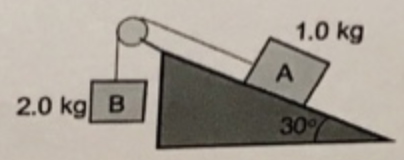# Problem: In the figure below, the pulley has negligible mass and is frictionless. The coefficient of static friction, μs, between block A and the incline in 0.430. Block A has a mass of 1.00 kg, block B has a mass of 2.00 kg and the angle of the incline is 30.0°.a) Draw a force diagram for block A. b) Draw a force diagram for block B. c) Is this situation in equilibrium or are the blocks moving? 1.0 kg 2.0 kg B 30 If the blocks are moving, which way are they moving?

###### FREE Expert Solution

(a)

The weight acts vertically downwards.

The reaction force acts perpendicularly to the surface/slope.

The kinetic friction force due to skidding acts parallel to the surface in the direction against the motion.

Force due to a gravitational acceleration component acts parallel to the surface directed downslope.

Tension acts upslope.

92% (481 ratings)###### Problem Details

In the figure below, the pulley has negligible mass and is frictionless. The coefficient of static friction, μs, between block A and the incline in 0.430. Block A has a mass of 1.00 kg, block B has a mass of 2.00 kg and the angle of the incline is 30.0°.a) Draw a force diagram for block A.

b) Draw a force diagram for block B.

c) Is this situation in equilibrium or are the blocks moving? 1.0 kg 2.0 kg B 30 If the blocks are moving, which way are they moving?